# RRB ALP 2018 Practice Test Papers | Arithmetic Questions (Day-72)

Dear Aspirants, Here we have given the Important RRB ALP & Technicians Exam 2018 Practice Test Papers. Candidates those who are preparing for RRB ALP 2018 can practice these Arithmetic Questions to get more confidence to Crack RRB 2018 Examination.

[WpProQuiz 3011]

Click “Start Quiz” to attend these Questions and view Solutions

1)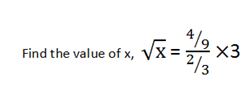a) 7

b) 6

c) 5

d) 4

2) Atul and Basu started a business with the investment cost of 15000 and 20000 respectively. After 6 months Atul increased his investment by 1/3rd of initial amount. What is the profit ratio for 2 years?

a) 8: 5

b) 5: 8

c) 3: 5

d)5: 3

In an Educational Intuition, Student studied in various departments. Total Number of Students is 8000.  Given pie chart represents the Percentage of studentsstudies in each department.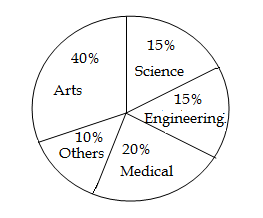3) How many students are there in Medical and Engineering Department?

a) 3000

b) 2800

c) 3500

d) 4500

4) What is the difference of sum of Arts and science students and sum of others and engineering students?

a) 3000

b) 2400

c) 3500

d) 2500

5) What is the ratio of Arts students and Engineering Student?

a) 5 : 2

b) 3 : 4

c) 8 : 3

d) 5 : 4

6) Number of Students studying in other department is what percentage of Medical students?

a) 180%

b) 50%

c) 100%

d) 200%

7) What is the average number of students studying in Arts, Science and Medical?

a) 2000

b) 2500

c) 3200

d) 3000

8) A number is added with its 3/4th value gives thrice the value of 7. Find the number.

a) 48

b) 12

c) 36

d) 24

9) Some workers can finish a work in 25 days. If 4 workers less, rest of the works can complete the work in 30 days. Find how many total workers are there initially?

a) 4

b) 8

c) 16

d) 24

10) What is 30 percentage of  50?

a) 12

b) 10

c) 15

d) 18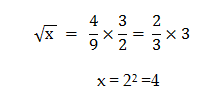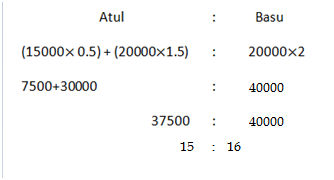Total Number of Students = 8000

% of Medical + Engineering student = 20 + 15 % = 35%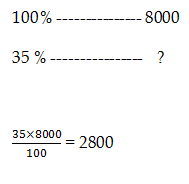Difference = (Arts + science) – (Others + Engineering)

= (40+15) % – (10+15) % = 55% – 25% = 30%

100————-8000

30 ————– ?

30/100×8000=2400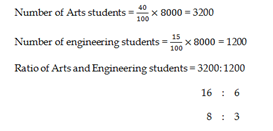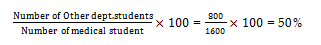Arts students = 3200

Science students = 1200

Medical students = 1600

Average = (3200+1200+1600)/3 = 6000/3 = 2000.

X + 3x/4 = 3*7= 21

(4x+3x)/4 = 21

4X+3x = 21 * 4

7x = 21* 4

X = 3*4 = 12

X workers do the work in 25 days

(x-4) workers do that work in 30 days

M1D1 = M2D2

25x = 30(x-4)

25 x = 30x – 120

5x = 120

X = 24